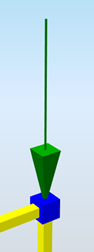# StructuralPointAction¶

Point force

Instances of StructuralPointAction define a point load applied to the node (StructuralPointConnection) and also the location of the point load on a 1D member (StructuralCurveMember).

The point force can be defined in three main directions (global or local coordinate system). Every point force has to be assigned to a load case.## Specification in the excel¶

Data type

Example / enum definition

Required

Description

Name

String

F3

yes

Human readable unique name of the force

Type

String

Standard

no

This property defines what the load is caused by, E.g. Standard, Wind, Snow, Self weight, Hoar Frost, Predefined, Plane Load, Water Pond, Water Pressure, Soil Pressure, Generated Water, Generated Soil

Direction

Enum

X

Y

Z

Vector

yes

Specifies the base direction of the load

X, Y, Z - action will be applied in one of these directions

Vector - size and direction calculated from vector

Force action

Enum

On beam

In node

yes

Specifies on which type of object the force acts

Reference node

String

N3

yes, if Force action = In node

The name of the reference node

Reference member

String

B1

yes, if Force action = On beam

The name of the reference member

Value [kN]

Double

-150

yes, if Direction = X,Y or Z

Specifies the size of the load in, acts in one direction

Vector (X;Y;Z) [kN]

String

(10;10;0)

yes, if Direction = Vector

Specifies the size of the load in , direction by vector

String

LC2

yes

The name of the load case to which the force belongs

Coordinate system

Enum

Global

Local

yes

Defines the co-ordinate system of the member in which the load is applied

For ” Local”, coordinate system is defined by node or 1D member

Origin

Enum

From start

From end

yes, if Force action = On beam

Specifies where the origin for the position co-ordinate measurement is

Coordinate definition

Enum

Absolute

Relative

yes, if Force action = On beam

Specifies the definition of the position. It may be absolute or relative

Position x [m]

Double

value in meters for Coordinate definition = Absolute

5.25

value in percentage for Coordinate definition = Relative

1.0

yes, if Force action = On beam

Defines the position of the load on the 1D member in local coordinate system in relative or in absolute coordinates [m]

Repeat (n)

Integer

0

yes, if Force action = On beam

Defines the number of forces acting on the beam. If the number is greater than 1, the forces are distributed uniformly over the 1D member

Delta x [m]

Double

0.3

yes, if Repeat (n) > 1

Defines the distance between forces acting on the 1D member in relative or in absolute coordinates [m]

(only applicable when ForceAction = OnBeam)

Id

String

39f238a5-01d0-45cf-a2eb-958170fd4f39

no

Unique attribute designation